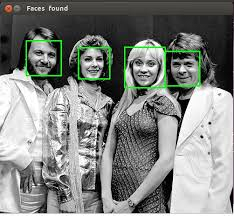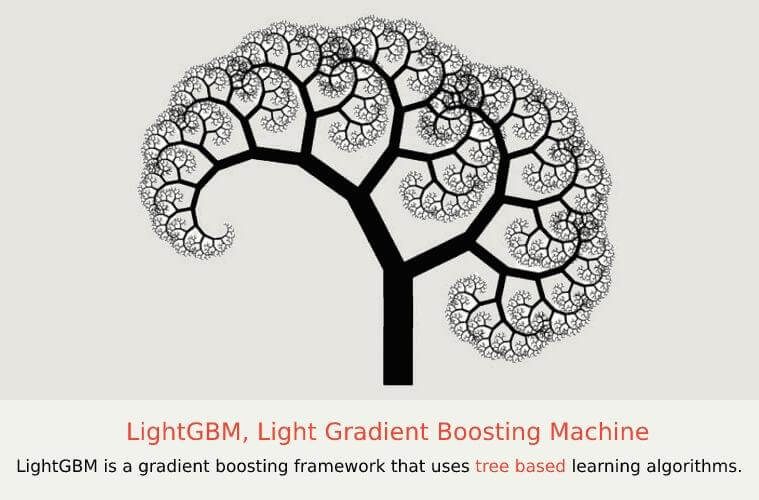• +91-9872993883
• +91-8283824812
• info@ris-ai.com

# Gender Classification using Python

In this article, we'll walk you through a Machine Learning project on Gender Classification Python.The Gender classification is gaining more and more attention, as gender contains significant information the social activities of men and women. The dataset we're working today is on human classification finding male and female. Today we are using different types of classifiers like:

1. Catboost Classifier
2. Light GBM Classifier
3. Random Forest Classifier
4. Decision Tree Classifier## Load important packages according to the classifiers need. ¶

In the below code , we are loading image processor because it identify the location of the defects from the image data and output this information to the end user. We've also used data loaders,when importing data, Data Loader reads, extracts, and loads data. Then we've specified paths that are loaded with dataset. Here we've used K-nn classifiers . K-nn classifiers find the nearest class that can be classified according to the input data we're provided with. The dataset today we've provided is about human classification on the basis of their gender (male or female). Here we have trained data with our own dataset and then we've tested it according to the classifier. Then we've used label encoding.

In :
import cv2
import os
import numpy as np
import pandas as pd
from sklearn.model_selection import train_test_split
from imutils import paths
from sklearn.neighbors import KNeighborsClassifier
from sklearn.metrics import classification_report
class ImgProcessor:
def __init__(self, width, height, inter=cv2.INTER_AREA):
self.width=width
self.height=height
self.inter=inter

def process(self, img):
return cv2.resize(img, (self.width, self.height), interpolation=self.inter)
def __init__(self, prepros=None):
self.prepros=prepros
if self.prepros is None:
self.prepros=[]

data = []
labels=[]

for(i, imgpath) in enumerate(imgpaths):
label=imgpath.split(os.path.sep)[-2]

if self.prepros is not None:
for p in self.prepros:
img=p.process(img)
data.append(img)
labels.append(label)

return (np.array(data), np.array(labels))
dataset_path = 'gender/valid'
neighbors = 3
jobs = 1
imgpaths=list(paths.list_images(dataset_path))
ip = ImgProcessor(32, 32)
data = data.reshape((data.shape,3072))
print("[INFO] features matrix: {:.1f}KB".format(data.nbytes / (1024)))
le = LabelEncoder()
labels = le.fit_transform(labels)
(Xtrain, Xtest, ytrain, ytest) = train_test_split(data, labels, test_size=0.25, random_state=40)
model = KNeighborsClassifier(n_neighbors=neighbors, n_jobs=jobs)
model.fit(Xtrain, ytrain)
print(classification_report(ytest, model.predict(Xtest), target_names=le.classes_))

Loading Images:
[INFO] features matrix: 600.0KB
precision    recall  f1-score   support

female       0.76      0.52      0.62        25
male       0.64      0.84      0.72        25

accuracy                           0.68        50
macro avg       0.70      0.68      0.67        50
weighted avg       0.70      0.68      0.67        50



### Using Cat boost classifier ¶

In the below code we are talking about the cat boost classifier. CatBoost is an algorithm for gradient boosting on decision trees. It gives accurate result on the given dataset. In the above program we've peformed that if predicted value is equal to tested value then it should give a result true and if the predicted value is greater or smaller then tested value it should give an output false. So to predict that value we've used cat boosting here.In :
from catboost import CatBoostClassifier
data = data.reshape((data.shape, 3072))
labels = le.fit_transform(labels)
from catboost import CatBoostClassifier
(X_train, X_test, y_train, y_test) = train_test_split(data, labels, test_size=0.25, random_state=40)
model_1 = CatBoostClassifier(iterations=2,learning_rate=0.1)
cbc = model_1.fit(X_train,y_train)
y_cbc = model_1.predict(X_test)
print(" Classifier\n",np.array(y_cbc == y_test)[:])
print('Percentage : ', 100*np.sum(y_cbc == y_test)/len(y_test))

0:	learn: 0.6444487	total: 357ms	remaining: 357ms
1:	learn: 0.6043677	total: 626ms	remaining: 0us
Classifier
[ True  True False  True False  True  True  True False  True  True  True
True  True False  True False  True  True  True  True  True  True  True
False False  True  True  True False  True  True  True  True  True  True
True  True False False  True  True  True  True  True  True  True False
True  True]
Percentage :  78.0


### Using Light gradient boosting classifier.¶

It is high performance gradient boosting framework based on Decision Tree Algorithms. It is used for classification and many other machine learning tasks. It gives accurate result on the given dataset. In the above program we've peformed that if predicted value is equal to tested value then it should give a result true and if the predicted value is greater or smaller then tested value it should give an output false. So to predict that value we've used light gbm boosting here.In :
import pandas as pd
import numpy as np
import lightgbm as lgb
from lightgbm import LGBMClassifier
from sklearn.metrics import accuracy_score
from sklearn.model_selection import train_test_split
data = data.reshape((data.shape, 3072))
labels = le.fit_transform(labels)
(X_train, X_test, y_train, y_test) = train_test_split(data, labels, test_size=0.25, random_state=40)
mg=LGBMClassifier()
model4= mg.fit(X_train, y_train)
y_pred = model4.predict(X_test)
print(" Classifier\n",np.array(y_pred == y_test)[:])
print('Percentage : ', 100*np.sum(y_pred == y_test)/len(y_test))

 Classifier
[ True False False  True  True False  True  True  True  True False  True
True  True False  True False  True  True  True  True  True  True False
False  True  True False  True False  True  True  True  True False  True
True False False  True  True  True  True  True  True  True  True  True
True  True]
Percentage :  74.0


### Using Random forest classifier. ¶

The random forest is a classification algorithm consisting of many decisions trees. It uses bagging and feature randomness when building each individual tree to try to create an uncorrelated forest of trees whose prediction by committee is more accurate than that of any individual tree. In the above program we've peformed that if predicted value is equal to tested value then it should give a result true and if the predicted value is greater or smaller then tested value it should give an output false.In :
import pandas as pd
import numpy as np
from sklearn.metrics import accuracy_score
from sklearn.ensemble import RandomForestClassifier
from sklearn.model_selection import train_test_split
data = data.reshape((data.shape, 3072))
labels = le.fit_transform(labels)
(X_train, X_test, y_train, y_test) = train_test_split(data, labels, test_size=0.25, random_state=40)
model2= RandomForestClassifier()
pr= model2.fit(X_train,y_train)
pre= pr.predict(X_test)
print(" Classifier\n",np.array(pre == y_test)[:])
print('Percentage : ', 100*np.sum(pre == y_test)/len(y_test))

 Classifier
[ True  True False  True  True False  True  True False  True False  True
True  True False  True  True False  True  True  True False  True  True
False  True  True  True  True False  True  True  True  True False False
True False  True  True  True  True  True  True  True  True  True  True
True  True]
Percentage :  76.0


#### Using decision tree classifier. ¶

Decision trees use multiple algorithms to decide to split a node into two or more sub-nodes. Each node in the tree specifies a test on an attribute, each branch descending from that node corresponds to one of the possible values for that attribute. In the above program we've peformed that if predicted value is equal to tested value then it should give a result true and if the predicted value is greater or smaller then tested value it should give an output false.In :
import pandas as pd
import numpy as np
from sklearn.metrics import accuracy_score
from sklearn.tree import DecisionTreeClassifier
from sklearn.model_selection import train_test_split
data = data.reshape((data.shape, 3072))
labels = le.fit_transform(labels)
(X_train, X_test, y_train, y_test) = train_test_split(data, labels, test_size=0.25, random_state=40)
model1= DecisionTreeClassifier()
dr= model1.fit(X_train,y_train)
pred= dr.predict(X_test)
print(" Classifier\n",np.array(pred == y_test)[:])
print('Percentage : ', 100*np.sum(pred == y_test)/len(y_test))

 Classifier
[ True False False False  True  True False  True  True False False  True
True  True  True False False  True  True  True  True  True  True  True
False False  True  True  True  True  True  True  True  True  True  True
True False  True  True False  True  True False  True  True  True  True
True  True]
Percentage :  74.0

##### Conclusion¶

From Gender Classification using Python article , we've learned that different classifiers works according to the user's need and most important thing every classifiers have their own compatibility to work on a dataset. In the above codes Cat Boost Classifier have the highest accuracy than other classifiers for classifying male and female dataset.